[Solved] original_keras_version = f.attrs[‘keras_version‘].decode(‘utf8‘)

windows system:
original_keras_version = f.attrs[‘keras_version’].decode(‘utf8’)
1. error:

original_keras_version = f.attrs['keras_version'].decode('utf8')

AttributeError: 'str' object has no attribute 'decode'

2. Cause analysis

When installing tensorflow, the default installed h5py is 3.1.0, and an error is reported because the TF you installed does not support an excessively high version of h5py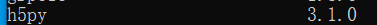3. Solutions

1. Uninstall h5py3 Version 1.0, installing h5py2.0 Version 10.0.2. Restart the compiler

pip install h5py==2.10.0

[Solved] Python Keras Error: AttributeError: ‘Sequential‘ object has no attribute ‘predict_classes‘

model = Sequential()
-
history = model.fit(X_train, y_train, batch_size = 256, epochs = 10, verbose = 2, validation_split = 0.2)
-
score, acc = model.evaluate(X_test, y_test,verbose=2, batch_size= 256)
print('test accuracy:', acc)
-
yhat_classes = model.predict_classes(X_test)

Cause of problem:

This predict was removed in tensorflow version 2.6_ Classes function.

Reference documents: https://keras.rstudio.com/reference/predict_proba.html#details

The following codes can be used:

predict_x=model.predict(X_test)
classes_x=np.argmax(predict_x,axis=1)

Or

You can also try tensorflow 2.5 or other versions to solve this problem.

Using tensorflow version 2.5, there may be the following warning messages:

tensorflow\python\keras\engine\sequential.py:455: UserWarning: model.predict_classes() is deprecated and will be removed after 2021-01-01. Please use instead:* np.argmax(model.predict(x), axis=-1), if your model does multi-class classification (e.g. if it uses a softmax last-layer activation).* (model.predict(x) > 0.5).astype("int32"), if your model does binary classification (e.g. if it uses a sigmoid last-layer activation).

How to Solve Keras calls plot_model error

1. Error information

When building the neural network model, you can call plot in keras_ The model module draws a schematic diagram of the model to facilitate the adjustment of the model structure:

from tensorflow.keras.models import Model
from tensorflow.keras.utils import plot_model
model = Model(dense_inputs+sparse_inputs, output_layer)
plot_model(model, "fm_model.png", show_shapes=True)

As a result, the following error messages appear:

(‘Failed to import pydot. You must pip install pydot and install graphviz (ht

Understand the error message: the installation is complete without pydot and graphviz packages

2. Solutions

2.1 installation of graphviz package

pip install graphviz

In Windows Environment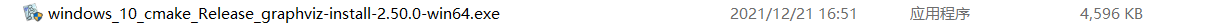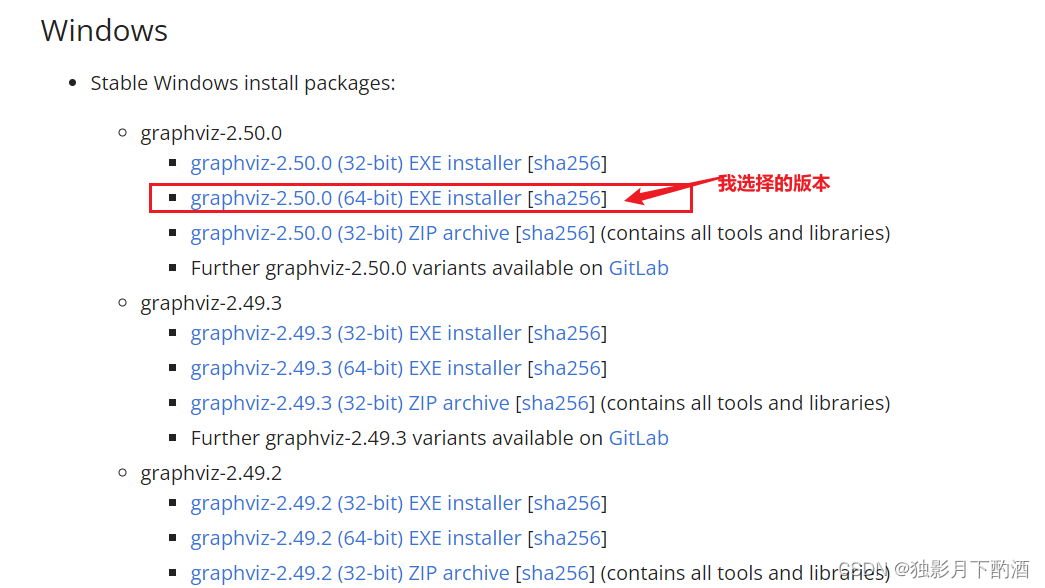2.3 configuring environment variables for graphviz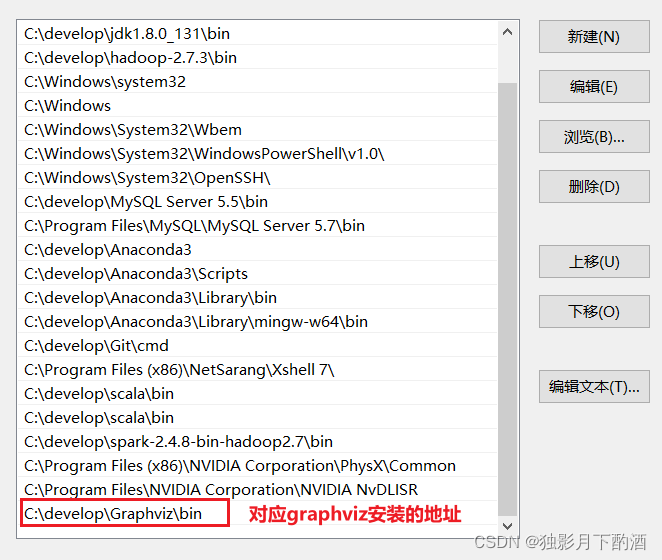2.4 installing pydot package

pip install pydot-ng

2.5 restart development tools

Restart the IDE or other development tools (Jupiter notebook) with immediate effect.

3. Summary

1. Installing pydot and graphviz packages directly according to the error message does not work

2. You need to go to the website to download the corresponding EXE file or zip file. After installation, specify the environment variables

3. Don’t forget to restart your ide or other development tools

Internalerror: GPU sync failed error (How to Solve)

1. Error reporting: (from Python deep learning p178-179)

When vscode runs the following code in Jupiter notebook, an error is reported: internalerror: GPU sync failed

from tensorflow.keras.models import Sequential
from tensorflow.keras import layers
from tensorflow.keras.optimizers import RMSprop

model = Sequential()

model.compile(optimizer=RMSprop(), loss='mae')
history = model.fit_generator(train_gen,
steps_per_epoch=500,
epochs=20,
validation_data=val_gen,
validation_steps=val_steps)

2. Solution:

(1) Don’t open too many ipynb file windows. There is only one running window left. Restart and there should be no problem.

(2) Some friends said that they might have something to do with the wallpaper engine. Just turn it off. I haven’t verified this yet.

However, I found that when the wallpaper engine dynamic desktop is displayed, the GPU utilization will increase sharply: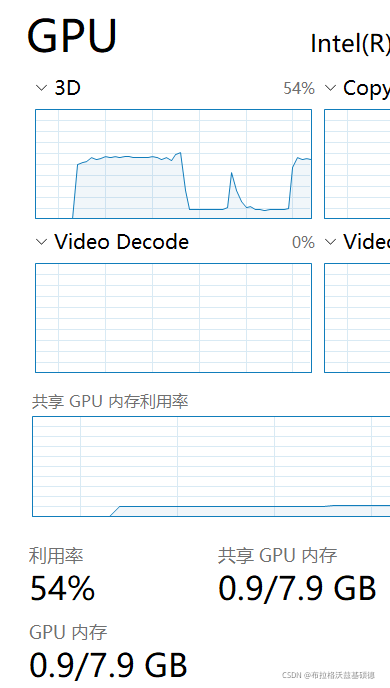[Solved] Keras Error: KeyError: ‘accuracy‘, KeyError: ‘val_acc‘, KeyError: ‘acc‘

Problem:
keyerror ‘ACC’ is reported when using keras

Reason:
this is a keras version problem. ACC and accuracy are intended to be the same, but different keras versions use different names, so they need to be replaced. val_ So is acc.

Solution:
Print history keyword
Print (history. History. Keys())
change the error part to the printed “K” and “V”“

[Solved] Tensorflow error or keras error and tf.keras error: oom video memory is insufficient

Hint: if you want to see a list of allocated tenants when oom happens, add Report_tensor_allocations_upon_oom to RunOptions for current allocation info.

Problem description

The problems encountered in today’s 50% off cross-validation and grid search are that the amount of data was too large or bitch_ It also occurs when the size is too large, as shown in the figure:
use the command: Watch – N 0.1 NVIDIA SMI in Linux to view the GPU usage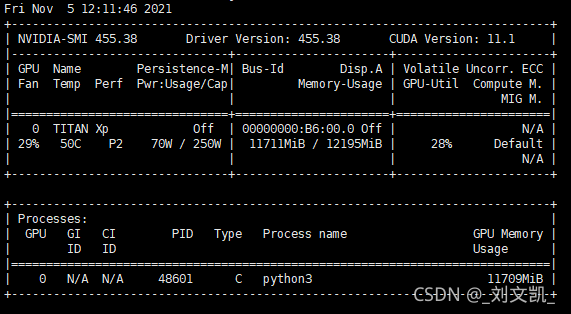reason

Due to the lack of video memory, but it is not the real lack of video memory, but because TensorFlow has eaten up the video memory, but there is no actual effective utilization. Therefore, the required video memory can be allocated to TensorFlow. (keras based on TensorFlow is also applicable)

Solution:

1. Set small pitch_Size, although it can be used, the indicator does not cure the root cause
2. Manually set the GPU. In train.py:

(1) in tensorflow
import tensorflow as tf
import os

os.environ["CUDA_VISIBLE_DEVICES"] = "0" Specify which GPU to use
config = tf.ConfigProto()
config.gpu_options.allow_growth = True # Allocate video memory on demand
config.gpu_options.per_process_gpu_memory_fraction = 0.4 # Maximum memory usage 40%
session = tf.Session(config=config)) # Create tensorflow session
...
(2) in keras
import tensorflow as tf
from keras.models import Sequential
import os
from keras.backend.tensorflow_backend import set_session ## Different from tf.keras

os.environ["CUDA_VISIBLE_DEVICES"] = "0"
config = tf.ConfigProto()
config.gpu_options.allow_growth = True  # Allocate video memory on demand
set_session(tf.Session(config=config)) # Pass the settings to keras

model = Sequential()
...
(3) in tf.keras
import tensorflow as tf
from tensorflow.keras.models import Sequential

import os
from tensorflow_core.python.keras.backend import set_session # Different from tf.keras

os.environ["CUDA_VISIBLE_DEVICES"] = "0"
config = tf.ConfigProto()
config.gpu_options.allow_growth = True  # Allocate video memory on demand
config.gpu_options.per_process_gpu_memory_fraction = 0.4 # use 40% of the maximum video memory
set_session(tf.Session(config=config)) # Pass the settings to tf.keras

model = Sequential()
...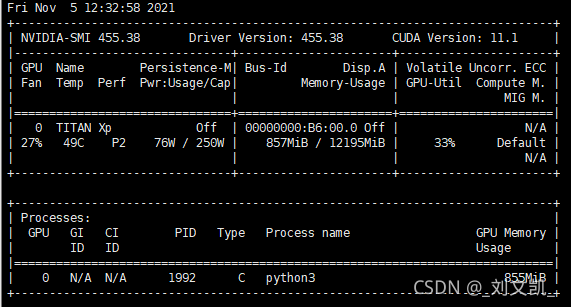Supplement:

model.fit(x_train，y_train，use_multiprocessing=True, workers=4) # Enable multithreading, using 4 CPUs

Empty session:

from tensorflow import keras
keras.backend.clear_session()

After emptying, you can continue to create a new session

Scenario:

at first, I suspected that I could not accurately locate the keras package of R, because I was using Anaconda to do other things, but later I saw other error reports and felt that they were not so complex… Later, I found that they were just simple and not installed properly… In short, the solution is as follows

Solution:

terminal input:

install.packages(tensorflow)
install_tensorflow()
library(tensorflow)
#Keras The R interface uses the TensorFlow backend engine.
# To install the core Keras library and TensorFlow backend, use the install_keras() function
install_keras()
library(keras)
# Run them all and then tune the package, if you have already done so, restart RStudio
-------------------------------------------
#Other solutions to this problem are as follows:
library(tensorflow)
library(keras)
use_condaenv("r-tensorflow")

ValueError: Negative dimension size caused by subtracting 2 from 1 for…

ValueError: Negative dimension size caused by subtracting 2 from 1 for ‘{{node max_pooling2d_1/MaxPool}} = MaxPoolT=DT_FLOAT, data_format=“NHWC”, explicit_paddings=[], ksize=[1, 2, 2, 1], padding=“VALID”, strides=[1, 2, 2, 1]’ with input shapes: [?,1,128,64].
channel Order problem
from keras import backend as K
K.set_image_data_format(‘channel_first’)
Done!

The function of flatten layer in deep learning

The official account of WeChatFlatten layer is implemented in Keras.layers.core . flatten() class.

effect:

Flatten layer is used to “flatten” the input, that is, to make the multi-dimensional input one-dimensional. It is often used in the transition from convolution layer to fully connected layer. Flatten does not affect the size of the batch.

example:

from keras.models import Sequential
from keras.layers.core import Flatten
from keras.layers.convolutional import Convolution2D
from keras.utils.vis_utils import plot_model

model = Sequential()
# now：model.output_shape==(None,64,32,32)

# now: model.output_shape==(None,65536)

plot_model(model, to_file='Flatten.png', show_shapes=True)

In order to better understand the function of flatten layer, I visualize this neural network, as shown in the figure below: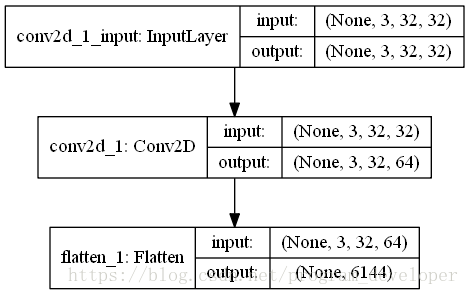Error in testing keras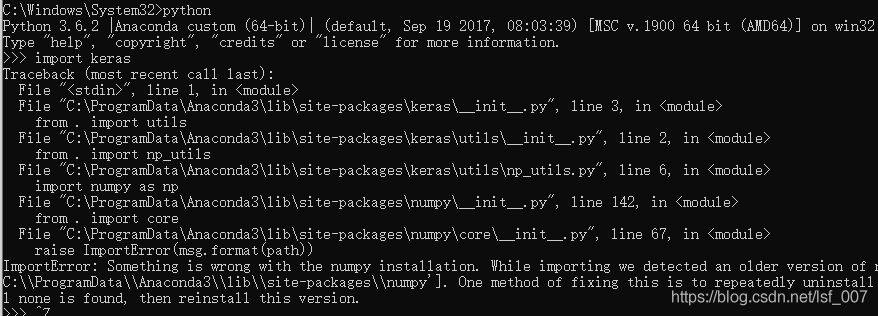nning Keras under Python is reporting an error — it tells me that one way to fix this problem is to uninstall Numpy repeatedly until I can’t find it, and then reinstall this version. Installation keras
no problem, baidu, a lot of reasons, all have been adjusted, still not solve the
I finally find a suitable way to
find their numpy location
below is I save position (for reference only, everyone is different)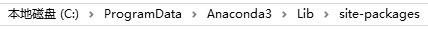where will use the red pen circle below are deleted, then reinstall the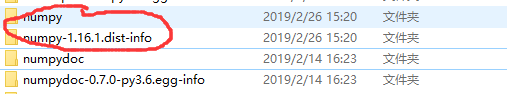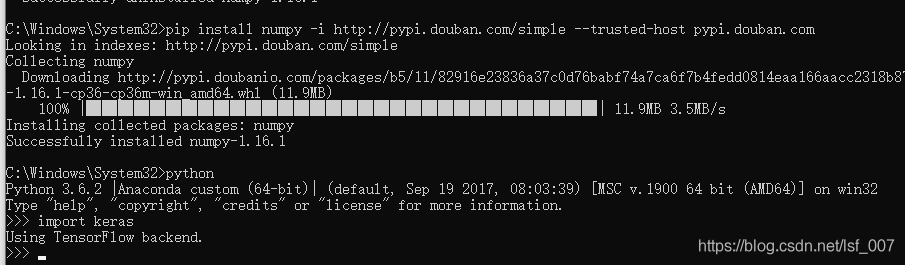this is solved!

Exception ignored in: bound method basesession__ del__ Of

Error message

Exception ignored in: <bound method BaseSession.__del__ of <tensorflow.python.client.session.Session object at 0x000000001AB286D8>>
Traceback (most recent call last):
File "python3.5.2\lib\site-packages\tensorflow\python\client\session.py", line xxx, in __del__
TypeError: 'NoneType' object is not callable

Reason: When the Python garbage collection mechanism collects the Session object, it finds that c_api_util or Tf_session has been collected, resulting in a null pointer. The following comment is the reason given when an exception is thrown in the source code of TensorFlow

# At shutdown, `c_api_util` or `tf_session` may have been garbage
# collected, causing the above method calls to fail. In this case,
# silently leak since the program is about to terminate anyway.

The solution
Method one:

import keras.backend as K

K.clear_session()

Method 2:

import gc

gc.collect()

reference
https://github.com/tensorflow/tensorflow/issues/3388
https://www.cnblogs.com/kaituorensheng/p/4449457.html

InternalError: Failed to create session. Error and solution

InternalError: Failed to create session. Errors and solutions
Introduction Error message solution

preface
This error occurred at the beginning of training Keras (using the TensorFlow as Backend) model.
Python version: 3.5.2
Keras version: 2.1.3
TensorFlow version: 1.9.0
Error message

InternalErrorTraceback (most recent call last)
< ipython-input-4-d4cc2ca313a3> in < module>
11 # fit network
— > 12 history = model.fit(X_train, y_train, epochs=3000, batch_size=16, validation_data=(x_test, y_test), verbose=2, shuffle=False)
13 #history = model.fit(X,y, epochs=3000, batch_size=16, Verbose = 2, shuffle = False)
14 # plot history
/usr/local/lib/python3.5/dist – packages/keras/models. Py fit in (self, x, y, batch_size, epochs, verbose, callbacks, validation_split, validation_data, shuffle, class_weight, sample_weight, initial_epoch, steps_per_epoch, validation_steps, **kwargs)
963 initial_epoch=initial_epoch,
964 steps_per_epoch=steps_per_epoch,
— > 965 validation_steps = validation_steps)

967 966 def the evaluate (self, x = None, y = None,
/usr/local/lib/python3.5/dist – packages/keras/engine/training. Py fit in (self, x, y, batch_size, epochs, verbose, callbacks, validation_split, validation_data, shuffle, class_weight, sample_weight, initial_epoch, steps_per_epoch, validation_steps, **kwargs)
1667 initial_epoch=initial_epoch,
1668 steps_per_epoch=steps_per_epoch,
-> 1669 validation_steps = validation_steps)

1671 1670 def the evaluate (self, x = None, y = None,
/usr/local/lib/python3.5/dist – packages/keras/engine/training. Py in _fit_loop (self, f, ins, out_labels, batch_size, epochs, verbose, callbacks, val_f, val_ins, shuffle, callback_metrics, initial_epoch, steps_per_epoch, validation_steps)
1204 ins_batch[i] = ins_batch[i].toarray()
1205
-> 1206 outs = f (ins_batch)
1207 if not isinstance (outs, a list) :
1208 outs = [outs]
/usr/local/lib/python3.5/dist – packages/keras/backend/tensorflow_backend py in the call (self, inputs)
2471 feed_dict[tensor] = value
2472 fetches = self.outputs + [self.updates_op] + self.fetches
-> 2473 session = get_session()
2474 updated = session.run(fetches=fetches, Feed_dict = feed_dict,
2475 * * self session_kwargs)
/usr/local/lib/python3.5/dist – packages/keras/backend/tensorflow_backend py in get_session ()
175 allow_soft_placement = True)
– & gt; 176 _SESSION = tf. The Session (config = config)
177 Session = _SESSION 178 if not _MANUAL_VAR_INIT:

/usr/local/lib/python3.5/dist – packages/tensorflow/python/client/Session. Py in init (self, Target, graph, config)
1561
1562 “”
-& gt; 1563 super(Session, self).init(target, graph, config=config)
1564 # NOTE(mrry): Create these on first __enter__ to avoid a reference cycle.
1565 self._default_graph_context_manager = None
/usr/local/lib/python3.5/dist – packages/tensorflow/python/client/session. Py in init (self, target, graph, the config).
631 if self _created_with_new_api:
632 # pylint: Disable = protected – access
– & gt; 633 self._session = tf_session.TF_NewSession(self._graph._c_graph, opts)
634 # pylint: enable=protected-access
635 else:
InternalError: Failed to create session.

The solution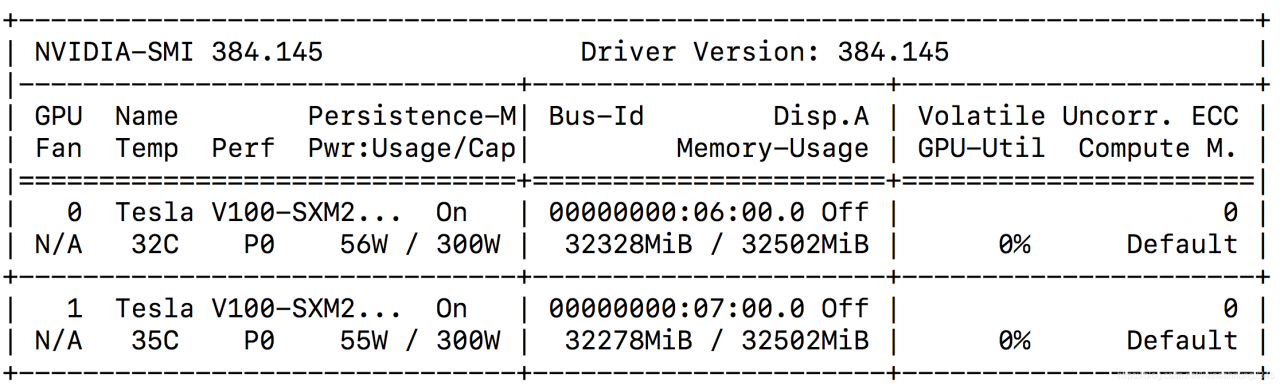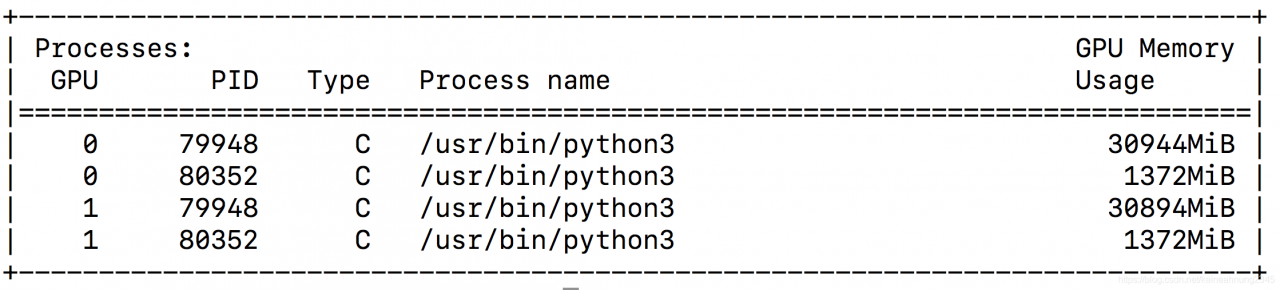found that GPU memory has been occupied by other programs.
try to close these programs, then reruns the code (no need to restart kernel) and find the problem solved!